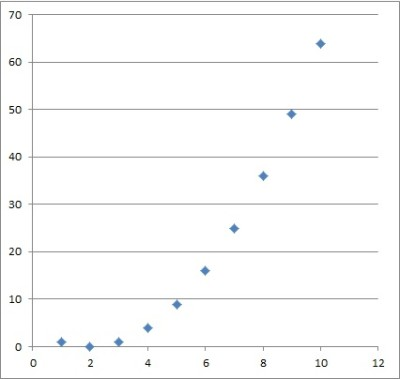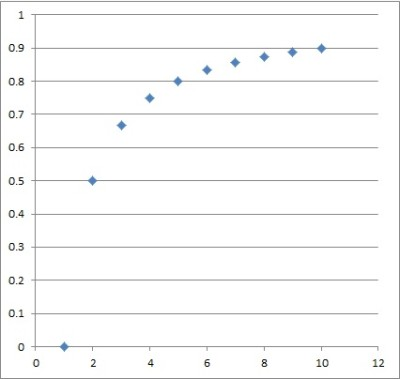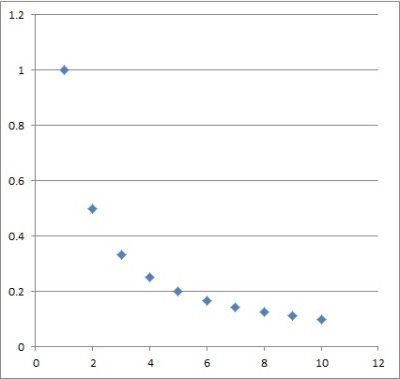# Increasing & Decreasing Sequences (5 Things You Should Know)

Increasing and decreasing sequences are important in mathematical analysis.  They help us to figure out whether a sequence converges and whether the corresponding sum converges.

So, when is a sequence increasing or decreasing?  A sequence of real numbers {ai} is increasing if am <= an for m < n, and decreasing if am >= an for m < n. A sequence can be increasing, decreasing, or neither, but it can never be both increasing & decreasing. An increasing sequence bounded above (or a decreasing sequence bounded below) converges.

Of course, we may also see strictly (monotonically) increasing or decreasing sequences, in which no two terms are the same.

In this article, we’ll talk about increasing and decreasing sequences and how to recognize them.  We’ll also look at some examples of both to make the concepts clear.

Let’s get started.

## Increasing & Decreasing Sequences

Remember that a sequence of numbers {ai} is a collection of numbers with a specific order.  The index i determines the order:

• i = 1 denotes a1, the first number in the sequence
• i = 2 denotes a2, the second number in the sequence
• i = n denotes an, the nth number in the sequence

A sequence may have repeated terms, although there are some types (monotonic sequences) that do not have repeated terms.

We can study how the terms progress to determine if the sequence is increasing, decreasing, or neither.  This can also help us to determine if the sequence converges or not.

### What Is An Increasing Sequence?

An increasing sequence is one where the next term is always greater than or equal to the previous term.  Using the sequence notation from before, we say:

• {ai} is an increasing sequence if a <= an for m < n

where the indices m and n are natural numbers (from the set 1, 2, 3, 4, …).

Another way to say this is with the following chain of inequalities:

• For an increasing sequence {ai}, a1 <= a2 <= a3 <= a4 <= …

If the terms are strictly increasing (no two terms are equal), then we say that:

• {ai} is a monotonically increasing sequence if a < an for m < n

Another way to say this is with the following chain of inequalities:

• For a monotonically increasing sequence {ai}, a1 < a2 < a3 < a4 < …

Let’s look at some examples of increasing sequences.

#### Example 1: An Increasing Sequence

Consider the sequence {ai} where ai­ = i2 – 3i.

The first several terms are -2, -2, 0, 4, 10, …

You can see the graph of the first several terms below:

We can see that this sequence is increasing just by looking at the terms.  However, we can also prove it as follows.

If m and n are indices (natural numbers) with m < n, then am = m2 – 3m and an = n2 – 3n .  This means that for any index greater than 3:

• am
• = m2 – 3m
• = m(m – 3)
• < n(m – 1)  [since m < n]
• < n(n – 1)  [since m < n, then m – 1 < n – 1]
• = n2 – n
• = an

This chain of inequalities proves that if m < n, then am <= an for any index greater than 3.

For the first three indices 1, 2, and 3, we can see that the sequence is increasing: -2, -2, 0.

However, this sequence is not monotonically increasing, since the first two terms are equal: a1 = -2 and a2 = -2.

#### Example 2: A Monotonically Increasing Sequence

Consider the sequence {ai} where ai­ = i.

The first several terms are 1, 2, 3, 4, 5, …

You can see the graph of the first several terms below:

We can see that this sequence is monotonically increasing just by looking at the terms.  However, we can also prove it as follows.

If m and n are indices (natural numbers) with m < n, then am = m and an = n.  Then am < an.

### What Is A Decreasing Sequence?

A decreasing sequence is one where the next term is always less than or equal to the previous term.  Using the sequence notation from before, we say:

• {ai} is a decreasing sequence if a >= an for m < n

where the indices m and n are natural numbers (from the set 1, 2, 3, 4, …).

Another way to say this is with the following chain of inequalities:

• For a decreasing sequence {ai}, a1 >= a2 >= a3 >= a4 >= …

If the terms are strictly increasing (no two terms are equal), then we say that:

• {ai} is a monotonically increasing sequence if a < an for m < n

Another way to say this is with the following chain of inequalities:

• For a monotonically decreasing sequence {ai}, a1 > a2 > a3 > a4 > …

Let’s look at some examples of decreasing sequences.

#### Example 1: A Decreasing Sequence

Consider the sequence {ai} where ai­ = -2i2 + 6i.

The first several terms are 4, 4, 0, -8, -20, …

You can see the graph of the first several terms below:

We can see that this sequence is decreasing just by looking at the terms.  However, we can also prove it as follows.

If m and n are indices (natural numbers) with m < n, then am = -2m2 + 6m and an = -2n2 + 6n .  This means that for any index greater than 3:

• am
• = -2m2 + 6m
• = -2m(m – 3)
• > -2n(m – 3)  [since m < n, multiplying by -2 negative flips the inequality to -2m > -2n]
• > -2n(n – 3)  [since m < n, then m – 3 < n – 3, and –2n(m – 3) > -2n(n – 3)]
• = -2n2 + 6n
• = an

This chain of inequalities proves that if m < n, then am >= an for any index greater than 3.

For the first three indices 1, 2, and 3, we can see that the sequence is decreasing: 4, 4, 0.

However, this sequence is not monotonically decreasing, since the first two terms are equal: a1 = 4 and a2 = 4.

#### Example 2: A Monotonically Decreasing Sequence

Consider the sequence {ai} where ai­ = -i2.

The first several terms are -1, -4, -9, -16, -25, …

You can see the graph of the first several terms below:

We can see that this sequence is monotonically decreasing just by looking at the terms.  However, we can also prove it as follows.

If m and n are indices (natural numbers) with m < n, then am = -m2 and an = -n2.

For any positive integers m and n, if m < n, then m2 < n2.

If we multiply both sides of the inequality by -1, we also flip the inequality symbol to get –m2 > -n2.

So, am > an­ whenever m < n.

### Can A Sequence Be Both Increasing & Decreasing?

A sequence cannot be both increasing and decreasing except in one very specific case: when the sequence is constant.  Here is the proof.

Assume otherwise: that a sequence {ai} is both increasing and decreasing.

Since {ai} is increasing, then a1 <= a2 <= a3 <= a4 <= …

Since {ai} is decreasing, then a1 >= a2 >= a3 >= a4 >= …

Since ai <= ai+1 and ai >= ai+1, then ai = ai+1 for every index i.

This means that the entire sequence has the same value, a1.  It is a constant sequence.

Note: by the same reasoning above, a sequence cannot be both monotonically increasing and monotonically decreasing.

Also, a sequence can be neither increasing nor decreasing.  Let’s look at some examples.

#### Example 1: A Sequence That Is Neither Increasing Nor Decreasing

Consider the sequence {ai} where ai = (-1)n.

The first several terms are -1, 1, -1, 1, -1, 1 … (note that this is called an alternating sequence, since the terms alternate between positive and negative).

You can see the graph of the first several terms below:

We can see that this sequence is neither increasing nor decreasing just by looking at the terms.

First, we see an increase from a1 to a2, since -1 < 1.

Next, we see a decrease from a2 to a3, since 1 > -1.

This continues on forever, so the sequence is neither increasing nor decreasing.

#### Example 2: A Sequence That Is Neither Increasing Nor Decreasing

Consider the sequence {ai} where ai = i2 – 4i + 4.

The first several terms are 1, 0, 1, 4, 9, …

You can see the graph of the first several terms below:This is the graph of the sequence {ai} where ai­ = i2 – 4i + 4.

We can see that this sequence is neither increasing nor decreasing just by looking at the terms.

First, we see a decrease from a1 to a2, since -1 > 0.

Next, we see an increase from a2 to a3, since 0 < 1.

Since we see both an increase and a decrease, the sequence is neither increasing nor decreasing.

Note: if we consider the subsequence {ai} for i > 1, then we have an increasing sequence.

### Can An Increasing Sequence Converge?

An increasing sequence can converge, but it must be bounded above.

If L the least upper bound of a sequence {ai}, then the sequence converges to L.

Let’s look at some examples of increasing sequences.

#### Example 1: An Increasing Sequence That Converges

Consider the sequence {ai} where ai = (i – 1) / i.

The first several terms are 0, 1/2, 2/3, 3/4, 4/5, …

You can see the graph of the first several terms below:This is the graph of the increasing convergent sequence {ai} where ai = (i – 1) / i. It converges to 1.

We can see that this sequence is increasing just by looking at the terms.

We can also see that the sequence is bounded above by 1, as follows:

• ai
• = (i – 1) / i
• = i/i – 1/i
• = 1 – 1/i
• <= 1  [since -1/i is negative]

Since {ai} is increasing and bounded above, it converges to the least upper bound of the sequence.

In this case, the least upper bound is 1, so {ai} converges to 1.

#### Example 2: An Increasing Sequence That Does Not Converge

Consider the sequence {ai} where ai = i.

The first several terms are 1, 2, 3, 4, 5, …

You can see the graph of the first several terms below:

We can see that this sequence is increasing just by looking at the terms.

We can also see that the sequence is not bounded above, since the terms increase as the index i increases.

Since {ai} is not bounded above, it does not converge (it is divergent).

### Can A Decreasing Sequence Converge?

A decreasing sequence can converge, but it must be bounded below.

If U the greatest lower bound of a sequence {ai}, then the sequence converges to U.

Let’s look at some examples of decreasing sequences.

#### Example 1: A Decreasing Sequence That Converges

Consider the sequence {ai} where ai = 1/i.

The first several terms are 1, 1/2, 1/3, 1/4, 1/5, …

You can see the graph of the first several terms below:This is the graph of the decreasing convergent sequence {ai} where ai­ = 1/i. It converges to zero.

We can see that this sequence is decreasing just by looking at the terms.

We can also prove it as follows: if m < n, then 1/m > 1/n, meaning am > an.

We can also see that the sequence is bounded below by 0, as follows:

• ai
• = 1 / i
• >= 0  [since 1/i is positive for any natural number i]

Since {ai} is decreasing and bounded below, it converges to the greatest lower bound of the sequence.

In this case, the greatest lower bound is 0, so {ai} converges to 0.

#### Example 2: A Decreasing Sequence That Does Not Converge

Consider the sequence {ai} where ai = -i2.

The first several terms are -1, -4, -9,. -16, -25…

You can see the graph of the first several terms below:

We can see that this sequence is decreasing just by looking at the terms.

We can also see that the sequence is not bounded below, since the terms become more negative (larger absolute value) as the index i increases.

Since {ai} is not bounded below, it does not converge.

## Conclusion

Now you know a little more about increasing and decreasing sequences, including what they are and how to identify them.